# Lakhmir Singh & Manjit Kaur: Friction- 3 Notes | Study Science Class 8 - Class 8

## Class 8: Lakhmir Singh & Manjit Kaur: Friction- 3 Notes | Study Science Class 8 - Class 8

The document Lakhmir Singh & Manjit Kaur: Friction- 3 Notes | Study Science Class 8 - Class 8 is a part of the Class 8 Course Science Class 8.
All you need of Class 8 at this link: Class 8

Q.66.
(A) Define friction. What are the factors affecting friction? Explain with examples.

The force that opposes the motion of an object is called friction. Example, when we walk, friction acts in opposite direction we apply force to walk.
The factor affecting friction are:

• The weight of the object.
• The type of contact between the two surfaces.

(B) What is the cause of friction? Explain with the help of a labelled diagram.

The cause of friction is surface roughness and adhesion.
When we apply force, F on a book, the book slide (Fig A). Then acts a force of friction, f (Fig B)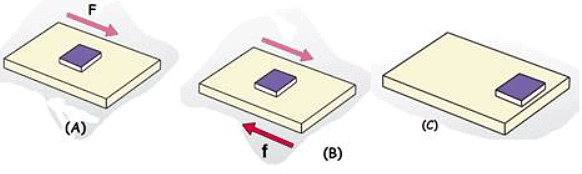The friction is produced due to the contact of the two surfaces of the table and the book. It acts in opposite direction of the force to stop it. And, we see after some distance, the book again comes to the rest (Fig C).

Q.67.
(A) Give examples to show that friction depends on the nature of two surfaces in contact.

The friction depends on the roughness as well as smoothness of the surface. If the surface is rough, then the surfaces in contact have more interlockings and the thus the friction is more. Thus, the object is not able to move easily due to more friction.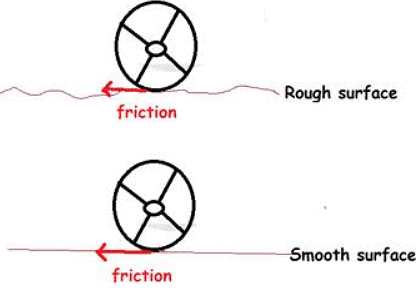If the surface is smooth, then the surfaces in contact have very less interlockings and the thus the friction is less. Thus, the object is able to move easily due to less friction.

(B) Give an example to show that friction depends on the force with which the two surfaces are pressed together.

Suppose, a person is pushing a heavy box, then he is applying say force F1.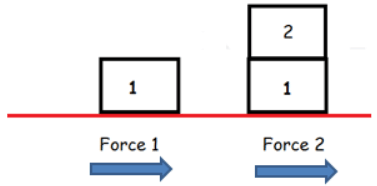Now if the same person pushes two boxes of same mass as the previous case. Then, in this case he will have to apply more force to overcome the larger friction as in the earlier case. Thus is because friction depends on the mass of the object. Heavier the mass, more the force is exerted by the object on the surface and thus more friction is produced.

Q.68.
(A) What is the difference between static friction and sliding friction? For’ a given pair of objects, which of the two is greater?

When the body is in stationary state or is at rest, the frictional forces acting on the body is known as static frictional forces.
But when the object is pushed and it moves, the friction that comes into action is sliding friction.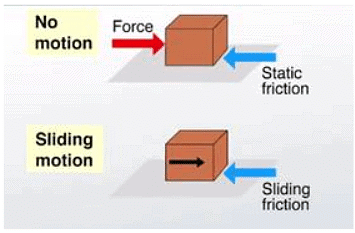The static friciton is greater than sliding friction because more force is required to remove the objectf rom rest state into dynamic state. Once the obejct comes into motion, it is easier to keep the object moving by applying comparetively lesser force.

(B) How can a very heavy machine be moved conveniently from one place to another in a factory? (No crane is available for this purpose).

A very heavy machine can be moved from one place to another with the help of wheels. Because then rolling friction comes into action and its value is the least among static and sliding friction.
The following diagram shows, how a heavy cylinder can be rolled easily.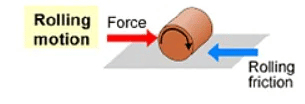Q.69.
(A) What is drag? Give two examples of a drag force.

The drag is the other name for fluid friction. It resists the motion of any object that travels in fluid.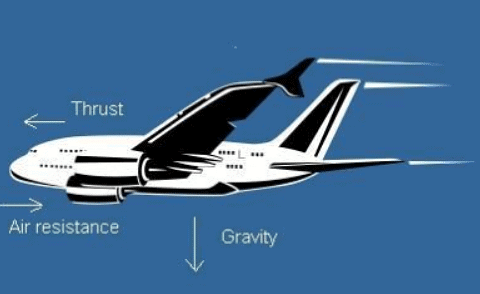Examples: 1. A boat travelling in water.
2. An aeroplane moving in the sky.

(B) How can you reduce the drag on something moving through the air?

The objects are built in special streamline shape to reduce the drag while moving in the air. Example, birds and Aeroplane have streamlined shapes.

Q.70.
(A) What is meant by ‘streamlined shape’? Name an object which usually has a streamlined shape.

A streamline shape is the one in which the body is pointed at the ends and broad-shaped in the middle.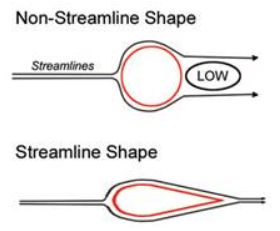Example : Speedboat has streamlined shape.

(B) Explain why, objects moving in fluids should have streamlined shape.

The objects moving in fluids have streamlined shape so as to reduce the air drag or fluid friction that opposes their motion in any fluid.

Multiple Choice Questions

Q.71. A boy runs his toy car on dry marble floor, wet marble floor, newspaper and towel spread on the floor. The force of friction acting on the car on different surfaces in increasing order will be :
(a) Wet marble floor, Dry marble floor, Newspaper, Towel
(b) Newspaper, Towel, Dry marble floor, Wet marble floor
(c) Towel, Newspaper, Dry marble floor, Wet marble floor
(d) Wet marble floor, Dry marble floor, Towel, Newspaper

As the roughness of the surface increases, the friction force increases.

Q.72. Four children were asked to arrange forces due to rolling, static and sliding frictions in a decreasing order. The correct arrangement is :
(a) Rolling, Static, Sliding
(b) Rolling, Sliding, Static
(c) Static, Sliding, Rolling
(d) Sliding, Static, Rolling

The static friction > Sliding Friction > Rolling friction.

Q.73. A big wooden box is being pushed on the ground from east to west direction. The force of friction due to ground will act on this box towards:
(a) north direction
(b) south direction
(c) east direction
(d) west direction

The force of friction acts opposite to the direction of motion.

Q.74. A spring balance can be used to measure
(i) Mass of an object
(ii) Force acting on an object
(iii) Density of an object
(iv) Weight of an object
(a) ii and ii
(b) ii and iii
(c) ii and iv
(d) only iv

A spring balance can measure both the force acting on it as well as the weight of the object.

Q.75. The friction between two surfaces does not depend on one of the following. This one is:
(a) amount of surface area of the two objects which is in contact with each other
(b) weight of the object which tends to move on the surface of other object
(c) degree of smoothness of surfaces of two objects in contact with each other
(d) degree of roughness of surfaces of two objects in contact with each other

Friction does not depend on the area of contact.

Q.76. If the sliding friction between two surfaces is found to be 8 N, then the static friction between these two surfaces is most likely to be:
(a) 5 N
(b) 10 N
(c) 4 N
(d) 2 N

The static friction is always greater than sliding friction.

Q.77. Which of the following is not an advantage of friction?
(a) it enables drawing to be made on paper
(b) it enables fallen things to be picked up
(c) it enables rubber pads to be rubbed off
(d) it enables vehicles to move on ground

Friction does wear and tear of the objects.

Q.78. Which of the following statements is incorrect ?
(a) static friction is greater than rolling friction
(b) sliding friction is less than rolling friction
(c) rolling friction is less than static friction
(d) static friction is greater than sliding friction

The static friction > Sliding Friction > Rolling friction.

Q.79. If the static friction between two surfaces X and Y is found to be 20 N, then the rolling friction between these two surfaces should most likely be :
(a) 25 N
(b) 20 N
(c) 5 N
(d) 50 N

The relationship between the three types of friction is:

The static friction > Sliding Friction > Rolling friction Thus the rolling friction will be less than 20N

Q.80. If the static friction between two surfaces P and Q is measured to be 50 N, then the sliding friction between these two surfaces is most likely to be :
(a) 75N
(b) 45N
(c) 55N
(d) 65N

The static friction > Sliding Friction > Rolling friction.

Q.81. Which of the following will produce the maximum friction?
(a) rubbing of sand paper on glazed paper
(b) rubbing of sand paper on glass table top
(c) rubbing of sand paper on aluminium frame
(d) rubbing of sand paper on sand paper

As the roughness of the surface increases, the friction increases.

Q.82. Four similar cars having exactly the same mass are running at the same speed on the same road when brakes are applied at the same time. The cars come to a stop after covering distances of 5 m, 5.5 m, 4.8 m and 5.2 m respectively. The friction between the brake pads and discs will be the maximum in the car which travels the distance of:
(a) 5 m
(b) 5.5 m
(c) 4.8 m
(d) 5.2 m

As the distance travelled is the least in 4.8 m , thus friction would have been the largest.

Q.83. The weight of an object can be measured by a:
(a)  beam balance
(b) analytical balance
(c) spring balance
(d) physical balance

The spring balance measures weight of an object.

Q.84. A book is lying on the horizontal table top. If we tilt the table a little, then the book starts sliding down slowly. This happens because:
(a) sliding friction is greater than static friction
(b) sliding friction is less than force of gravity
(c) static friction is greater than sliding friction
(d) force of gravity is less than sliding friction

The book starts sliding down slowly sliding slowly, because the sliding friction is less than force of gravity.

Q.85. A body shape which offers very little resistance to the flow of air (or water) around it is called:
(a) trimlined shape
(b) steamlined shape
(c) streaklined shape
(d) streamlined shape

A body shape which offers very little resistance to the flow of a fluid around it, is called streamlined shape.

Q.86. Which of the following should be used to reduce friction on a carrom board?
(a) a lubricating oil
(b) a dry lubricant
(c) a layer of grease
(d) a ball bearing

Using talcum powder to reduce the friction on carom board.

Q.87. Which of the following does not have a streamlined shape?
(a) Aeroplane
(b) boat
(c) bird
(d) bus

Bus is not streamlined shape. It is a cuboid shape.

Q.88. The frictional force exerted by a fluid is called:
(a) brag
(b) drab
(c) drag

The frictional force exerted by fluids is called drag force.

Q.89. A person has applied some mustard oil on his hands. Which of the following objects will become most difficult for him to hold in his hand?
(a) Earthen cup (kulhar)
(b) thermocol tumbler
(c) glass tumbler
(d) wooden cup

Mustard oil reduces the friction on his hands. So, Glass tumbler will be most difficult to handle.

Q.90. Ball bearing is a device which usually converts:
(a) rolling friction into sliding friction
(b) static friction into sliding friction
(c) sliding friction into rolling friction
(d) rolling friction into static friction

Because rolling friction allows to move heavier objects from one place to other easily.

High Order Thinking Skills

Q.91. When a pencil cell is released from a certain point on an inclined wooden board, it travels a distance of 35 cm on floor A before it comes to rest. When the same pencil cell is released from the same point on the same inclined board, it travels a distance of 20 cm on floor B before coming to rest. Which floor, A or B, offers greater friction? Give reason for your answer.

Floor B offers greater friction because it stops the moving pencil cell stop at a lesser distance of 20 cm on the floor. More the friction, more the surface is rough.

Q.92. A car is moving towards North. What will be the direction of force of friction acting on this car due to surface of road ?

The direction of force of friction acting on this car due to surface of road is along the south direction i.e. opposite to the direction of motion.

Q.93. You spill a bucket of soapy water on a marble floor accidently. Would it make easier or more difficult for you to walk on the floor? Why?

It will make more difficult to walk on the floor. As soapy solution decreases the friction on the floor.

Q.94. What kind of friction comes into play:
(a) when a block of wood kept on table moves slowly ?

Sliding friction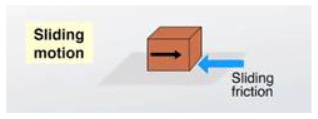(b) when a block of wood kept on table just tends to move (or slip)?

Static friction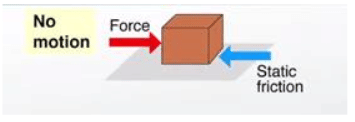(c) when a block of wood kept on cylindrical iron rods moves?

Rolling friction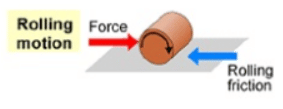Q.95. Explain why, it is easier to drag a mat on floor when nobody is sitting on it but much more difficult to drag the same mat when a person is sitting on it.

When no person is sitting on the mat, then the mat and floor are not pressed together.Thus, there is no friction.
But, when a person is sitting on the mat.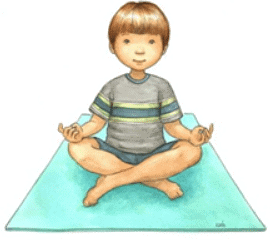Then due to the weight of the person, the mat and floor are pressed together harder, increasing the friction too much. Thus, then it is very difficult to drag the person.

The document Lakhmir Singh & Manjit Kaur: Friction- 3 Notes | Study Science Class 8 - Class 8 is a part of the Class 8 Course Science Class 8.
All you need of Class 8 at this link: Class 8Use Code STAYHOME200 and get INR 200 additional OFF

## Science Class 8

146 videos|352 docs|81 tests

Track your progress, build streaks, highlight & save important lessons and more!

,

,

,

,

,

,

,

,

,

,

,

,

,

,

,

,

,

,

,

,

,

;